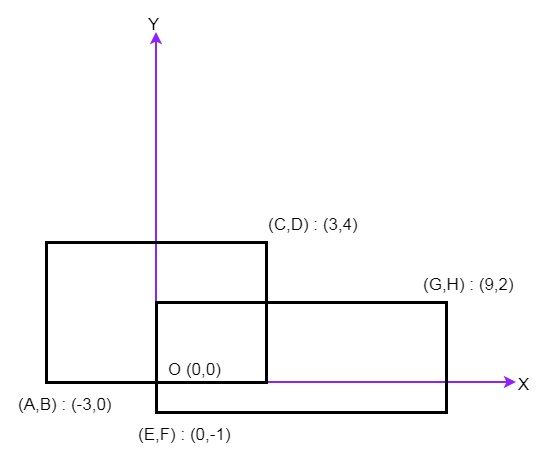# Program to find total area covered by two rectangles in Python

Suppose we want to find the total area covered by two rectilinear rectangles in a 2D plane. Here each rectangle is defined by its bottom left corner and top right corner as shown in the figure.To solve this, we will follow these steps −

• width_1 := |C-A|, height_1 := |D-B|
• width_2 := |G-E|, height_2 := |H-F|
• area := width_1*height_1 + width_2*height_2
• if (G<A) or (E>C) or (F>D) or (H<B), then
• return area
• otherwise,
• p := maximum of A, E
• q := maximum of B, F
• r := minimum of C, G
• s := minimum of D, H
• width_3 := |r-p|
• height_3 := |s-q|
• return area - (width_3*height_3)

## Example

Let us see the following implementation to get better understanding −

def solve(A, B, C, D, E, F, G, H):
width_1 = abs(C-A)
height_1 = abs(D-B)

width_2 = abs(G-E)
height_2 = abs(H-F)

area = width_1*height_1 + width_2*height_2

if (G<A) or (E>C) or (F>D) or (H<B):
return area
else:
p = max(A,E)
q = max(B,F)
r = min(C,G)
s = min(D,H)

width_3 = abs(r-p)
height_3 = abs(s-q)

return area - (width_3*height_3)

A = -3
B = 0
C = 3
D = 4
E = 0
F = -1
G = 9
H = 2
print(solve(A, B, C, D, E, F, G, H))

## Input

-3, 0, 3, 4, 0, -1, 9, 2


## Output

45